# Sketch and Constrain Feature Graphic Inputs - Intergraph Smart 3D - Reference Data

## Intergraph Smart 3D 2D Symbols

PPMProductFamily
3D Design and Visualization
PPMProduct
Intergraph Smart 3D
PPMCategory
Reference Data
Version_S3D
12.1(2019)
Version_SPEM_custom
9.0.0
Version_SSK
10.0(2018)

Certain feature types require graphic inputs; others do not. Sketched features do not require that graphic inputs be defined. Edge features always require graphic inputs. Most corner features will require graphic inputs while certain corner features can be created like sketched features, without graphic inputs. See Creating Features for details.

Edge Feature Inputs

Edge features require two graphic input elements; a point and a B-spline curve. The graphic inputs must be defined according to the following rules:

• The point MUST be coincident with the (0,0) origin of the 2D Symbols file.

• The curve representing the edge MUST be a B-spline curve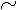. The curve need not include the (0,0) point in its definition, but it should pass near the origin point and be approximately horizontal.

• The curve and the point must be locked using the Lock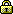command on the Relationship toolbar.

• The point and the curve do not need to be constrained together.

• The material of the part is located above the curve.

• The majority of the feature shape must be drawn above the input curve in 2D Symbols (on the positive side of the V-axis).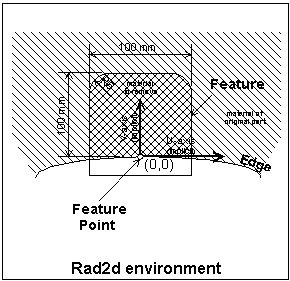1. Make the Input Layer active.

2. Place a point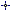at the (0,0) origin.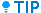The point is easier to find for later reference if you place a small circle centered on the point.

3. Create the curve approximately parallel to the +X axis, such that the (0,0) point is near the midpoint of the curve.

4. Lockthe point and the curve at its midpoint.

5. Delete the circle created in Step 2.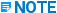The point defined as the symbol origin does not need to be located on the input curve. When the edge feature is placed in the model, it maps this symbol origin point to the placement point located on the edge of the 3D part. This point defines the location of the feature symbol relative to the 3D edge of the part. The symbol is oriented such that the symbol U-axis is tangent to the part edge at the placement point and the symbol V-axis is normal to the part edge at the placement point.

Corner Feature Inputs

Corner features require two graphic input curves be defined to represent the two 3D edges that intersect to define the corner of the part. To make the symbol as flexible as possible, the graphic inputs should be defined as B-spline curves. These graphic inputs will serve as the U and V axes of the symbol. The graphic inputs must be defined according to the following rules:

• B-spline curves should be defined using three or four points.

• The two B-splines curves forming the U- and V-axes of the symbol must intersect near the (0,0) point of the symbol file.

• The B-spline curve forming the U-axis should be approximately parallel to the +X 2D Symbols axis.

• The B-spline curve forming the V-axis should be approximately parallel to the +Y 2D Symbols axis.

• These two B-spline curves do not need to share a common endpoint.

• These two B-spline curves should not be constrained together.

• The symbol geometry must be drawn in the (+U,+V) quadrant of the coordinate axes defined by these two curves.

• All B-spline curves serving as graphic inputs must be locked at their midpoints.

1. Make the Input Layer active.

2. Locate the (0,0) point by placing a pointat (0,0).The point is easier to find for later reference if you place a small circle centered on the point.

3. Create the first curve approximately parallel to the +X axis, beginning just to the left of the (0,0) point and passing as close as possible to it.

4. Create the next curve approximately parallel to the +Y axis, beginning just below the (0,0) point and passing as close as possible to it.

5. Lock the curves at their midpoints.

6. Delete the (0,0) point and circle created in Step 2.